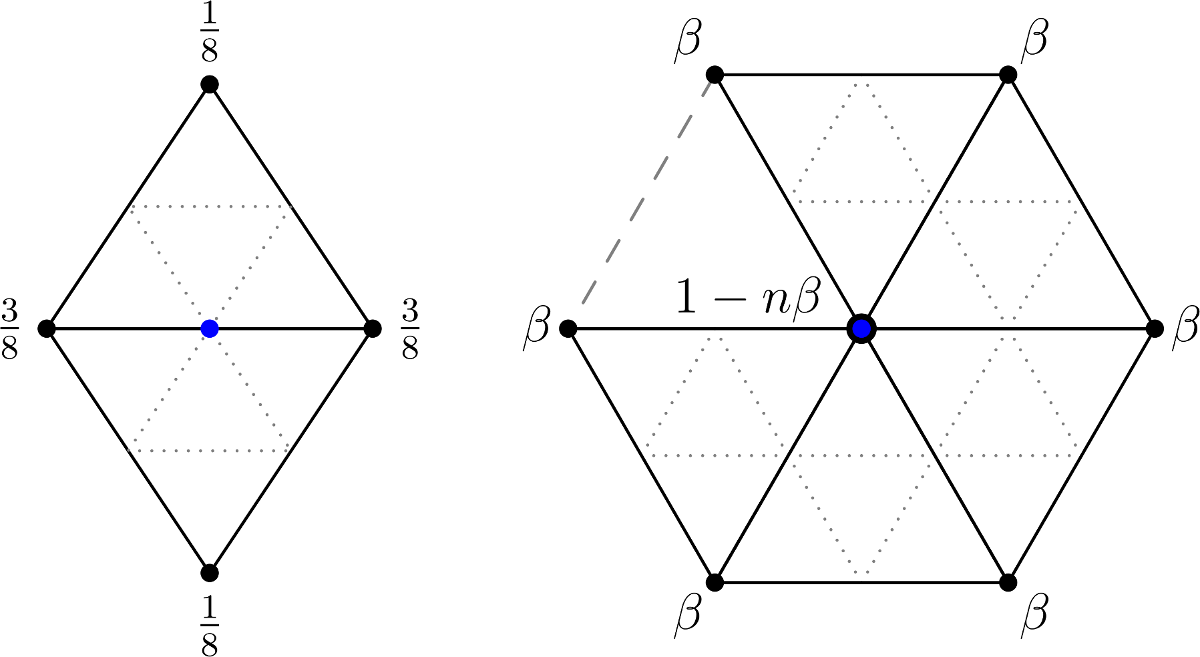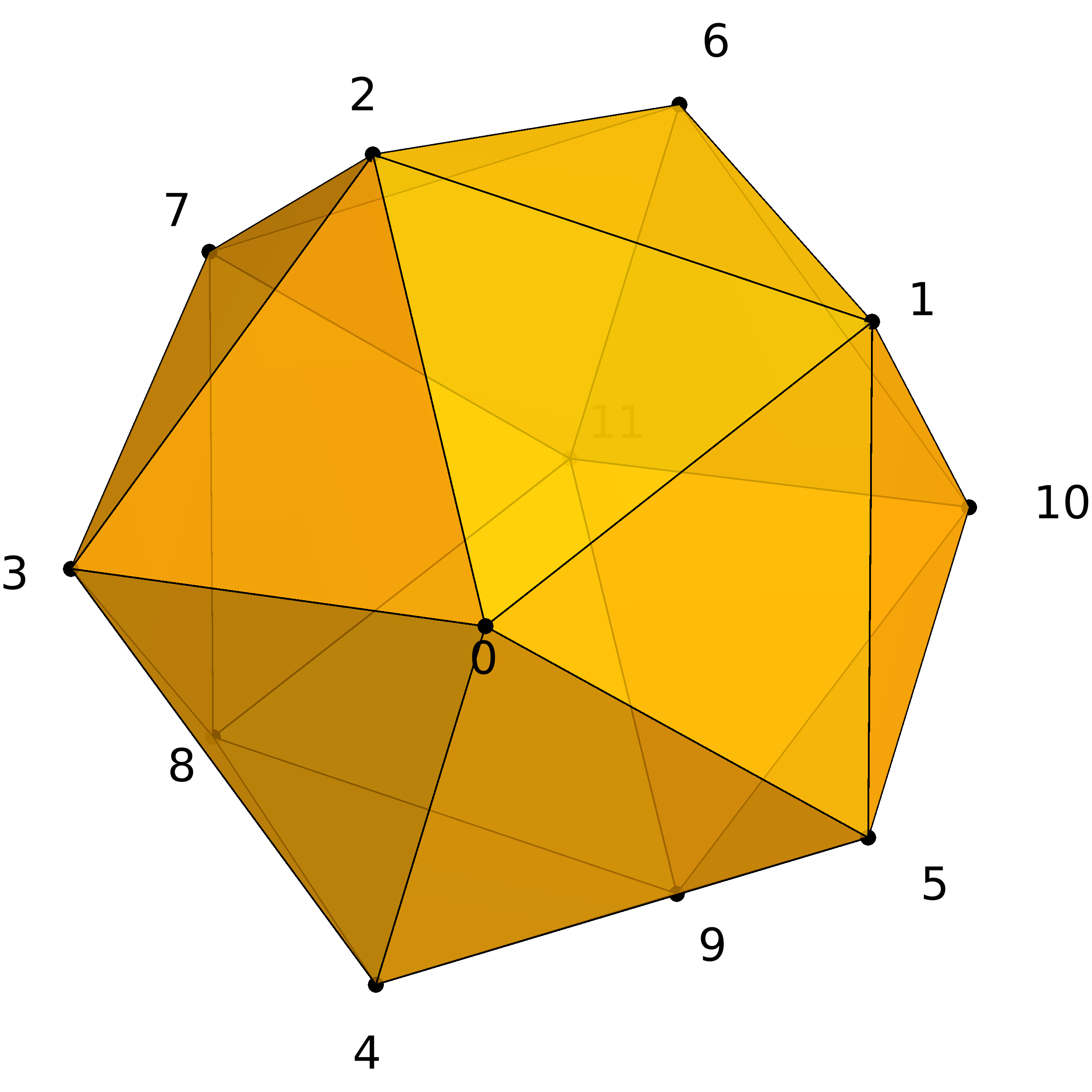TP9 submission deadline is Thursday, 13/04/2017 at 23:59. The TP on Friday 14/04 will start at 13:30 (instead of 15:15).

# TP9 : Subdivision Surfaces on Triangle Meshes

7 April 2016 in

## Subdivision surfaces

Mesh subdivision is a powerful tool, extensively used in modern geometric modeling. Unlike B-spline subdivision you implemented in TP8, subdivision surfaces are not limited to rectangular topology; in fact they provide the means for representing surfaces of arbitrary topology.

Almost every current animated movie uses subdivision surfaces to some extent. Even though their mathematical foundations date back to 1978, Pixar’s short Geri’s Game (1997) is considered to be the first ‘real’ application.Geri’s game, an Academy award-winning short movie by Pixar.

## Loop subdivision scheme

Today, you’ll be implementing the Loop subdivision scheme for triangle meshes. Like every surface subdivision scheme, it consists of two general steps:

1. Split the topology; each triangle is replaced by four smaller triangles.
2. Compute the new vertex positions from the old positions.

To compute the new geometry, two sets of vertices are recognized, each with its own rules: new edge midpoints and old vertices. Subdivision masks for both groups are visualised below.Edge and vertex masks for the Loop subdivision scheme.

The $n$ in the vertex mask denotes the number of neighbours. The original definition of $\beta$ was introduced by Charles Loop in his master’s thesis (1987):

Joe Warren proposed using the simplified weights (1995):

## Implementation

To represent a triangle mesh with arbitrary topology, we use two matrices.

• geometry VV[i] is the position of the vertex i.
• topology FF[j] are the indices of the three vertices in triangle j.

### Example: sphere

V = [
0.0000  -1.0000   0.0000
0.7236  -0.4472   0.5257
-0.2764  -0.4472   0.8506
-0.8944  -0.4472   0.0000
-0.2764  -0.4472  -0.8506
0.7236  -0.4472  -0.5257
0.2764   0.4472   0.8506
-0.7236   0.4472   0.5257
-0.7236   0.4472  -0.5257
0.2764   0.4472  -0.8506
0.8944   0.4472   0.0000
0.0000   1.0000   0.0000
];

F = [
0  1  2
1  0  5
0  2  3
0  3  4
0  4  5
1  5 10
2  1  6
3  2  7
4  3  8
5  4  9
1 10  6
2  6  7
3  7  8
4  8  9
5  9 10
6 10 11
7  6 11
8  7 11
9  8 11
10  9 11
];Four iterations of the Loop scheme on the sphere.

Our mesh is represented in a matrix form. To perform the topological step of the subdivision (replace each triangle by four smaller triangles), we will construct an edge matrix E with 6 columns and one row for each old face. The even columns 0,2,4 will contain the old vertices, while the odd columns 1,3,5 will store the newly inserted midpoints. All odd columns initially contain -1. We then progress through this matrix and at each iteration, we ‘subdivide’ one edge – we just need to make sure all instances of this edge are modified at the same time by assigning the same midpoint index.

Take for instance the face matrix of a tetrahedron :

3  1  0
3  2  1
3  0  2
2  0  1


We will look at the construction of the corresponding edge matrix. We start with

3 -1  1 -1  0 -1
3 -1  2 -1  1 -1
3 -1  0 -1  2 -1
2 -1  0 -1  1 -1


Now, we look at the first midpoint which has not yet been set, in this case 3 -1 1. This corresponds to the edge 3 1. The next midpoint will have index 4: we need to make sure to change midpoints for both instances of the edge 3 1 in the matrix E. The other one is in row 2:

3  4  1 -1  0 -1
3 -1  2 -1  1  4
3 -1  0 -1  2 -1
2 -1  0 -1  1 -1


Changing the next ‘free’ midpoint 1 -1 0 we get

3  4  1  5  0 -1
3 -1  2 -1  1  4
3 -1  0 -1  2 -1
2 -1  0  5  1 -1


Changing the next ‘free’ midpoint 0 -1 3 we get

3  4  1  5  0  6
3 -1  2 -1  1  4
3  6  0 -1  2 -1
2 -1  0  5  1 -1


…and so on until we change the last ‘free’ midpoint:

3  4  1  5  0  6
3  7  2  8  1  4
3  6  0  9  2  7
2  9  0  5  1  8


## ToDo

1. Implement the computation of new geometry in the Loop subdivision scheme using the original weights $\beta$.
2. Use the simplified weights (Warren) and compare the results.
3. Subdivide the sphere and cube five times. Which surface is closer to the real sphere? Why?

## Test surfaces

This is the list of all provided test surfaces, with vertex and face counts.

Don’t apply too many iterations on surfaces with more faces, as the number of faces grows exponentially. Take the horse mesh with 1000 faces for instance: we get 4000 faces after one iteration, 16000 after two, and 64000 after three.

In general, three iterations are sufficient. For the sphere and cube, up to five iterations should be ok.

#V #F
tetra 4 4
cube 8 12
sphere 12 20
testsurf 69 134
bumpycube 201 398
cow 278 552